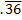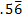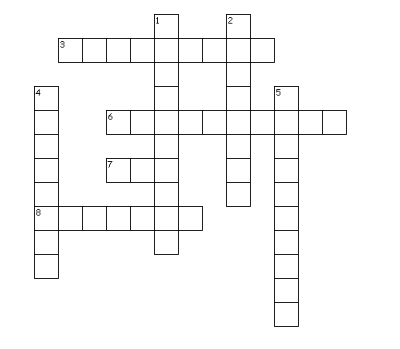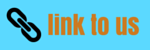# Class 9 Maths Important Questions for Number System

Notes Ncert Solutions Assignments Videos Revision sheet

Here we are trying to give the Class 9 Maths Important Questions for Number System. It tests the basic concepts and at the same makes the student comfortable with the questions

Question 1 Represent each number on number line 8/3 , 1.3 , -24 , 23/6
Question 2 Find a rational number lying between (i) 0.75 and 1.2 (ii) -3/4 and-2/5
Question 3 Insert six rational numbers. between 3 and 4
Question 4 Insert 16 rational numbers. between 2.1 and 2.2
Question 5 Express 0.9999999……. as a fraction in simplest form
Question 6  Locate the number √5 , √10 , √17  on Number line
Question 7)  Find which of the variables x, y, z and u represent rational numbers and which irrational numbers:
(i) x2 = 11 (ii) y2 = 36 (iii) z2 = .04 (iv) u2  = 19/9

Question 8)  Find an irrational number between 1/7 and 2/7
Question 9) Which is of these is the rational number between √2  and √3
(i) (√2  + √3)/2
(ii) 1.5
(iii) 1.8
(iv) (√2 . √3)/2

Question 10)
Expressandin the simplest form of rational no

Question 11)
Cross-word PuzzleAcross
3. rational numbers have only two choices- either they are terminating or non-terminating ________
6. The First person to discover the numbers which were not rational
7. The number (√3 -1)(√3 +1) is
8. Counting Number are called
Down
1. Non-terminating non recurring decimal expression
2. The set of positive and negative number is
4. Number of the form p/q
5. Who was the first to compute digits in the decimal expansion of π(Greek genius )